# Using the xtractomatic routines

## Introduction

`xtractomatic` is an R package developed to subset and extract satellite and other oceanographic related data from a remote server. The program can extract data for a moving point in time along a user-supplied set of longitude, latitude and time points; in a 3D bounding box; or within a polygon (through time). The `xtractomatic` functions were originally developed for the marine biology tagging community, to match up environmental data available from satellites (sea-surface temperature, sea-surface chlorophyll, sea-surface height, sea-surface salinity, vector winds) to track data from various tagged animals or shiptracks (`xtracto`). The package has since been extended to include the routines that extract data a 3D bounding box (`xtracto_3D`) or within a polygon (`xtractogon`). The `xtractomatic` package accesses data that are served through the ERDDAP (Environmental Research Division Data Access Program) server at the NOAA/SWFSC Environmental Research Division in Santa Cruz, California. The ERDDAP server can also be directly accessed at http://coastwatch.pfeg.noaa.gov/erddap. ERDDAP is a simple to use yet powerful web data service developed by Bob Simons.

This is the third version of `xtractomatic`. The original version was created by Dave Foley for Matlab and was modified for R by Cindy Bessey and Dave Foley. That version used a precurser to ERDDAP called BobDAP. BobDAP was less flexible and provided access to fewer datasets than does ERDDAP. The second version of xtractomatic was written by Roy Mendelssohn to use ERDDAP rather than BobDAP. This version, also written by Roy Mendelssohn, has many improvements including access to many more datasets, improved speed, better error checking, and crude search capabilites, and is the first version as a true R package.

### If you have used xtractomatic before

If you have existing R code that uses older versions of the `xtractomatic` functions, the code will likely require some simple and straightforward modifications to be used with this version. See the section What has Changed and Steps Going Forward

### The Main xtractomatic functions

There are three main data extraction functions in the `xtractomatic` package:

• `xtracto <- function(xpos, ypos, tpos, dtype, xlen, ylen, verbose=FALSE)`

• `xtracto_3D <- function(xpos, ypos, tpos, dtype, verbose=FALSE)`

• `xtractogon <- function(xpos, ypos, tpos, dtype, verbose=FALSE)`

There also are two information functions in the `xtractomatic` package:

• `searchData <- function(searchList=list(list("varname","chl")))`

• `getInfo <- function(dtype)`

The `dtype` parameter in the data extraction routines specifies a combination of which dataset on the ERDDAP server to access, and as well as which parameter from that dataset. The first sections demonstrate how to use these functions in realistic settings. The Selecting a dataset section shows how to find the `dtype` or other parameters of interest from the available datasets.

## Setting up

`xtractomatic` uses the `httr`, `ncdf4` and `sp` packages, and these packages must be installed first or `xtractomatic` will fail to install (the Windows version of `ncdf4` is not available through CRAN because of some quirks in CRAN policy, but it can be obtained at http://cirrus.ucsd.edu/~pierce/ncdf/).

``````install.packages("httr", dependencies = TRUE)
install.packages("ncdf4",dependencies = TRUE)
install.packages("sp", dependencies = TRUE)``````

The `xtractomatic` package is available from CRAN and can be installed by:

``install.packages("xtractomatic")``

or the development version is available from [Github](https://github.com/rmendels/xtractomatic and can be installed from Github,

``````install.packages("devtools")
devtools::install_github("rmendels/xtractomatic")``````

Once installed, to use `xtractomatic` do

``library("xtractomatic")``

If the other libraries (`httr`, `ncdf4`, and `sp`) have been installed they will be found and do not need to be explicitly loaded.

### Using the R code examples

Besides the `xtractomatic` packages, the examples below depend on `ggplot2`, `ggfortify`, `lubridate`, `mapdata`, `RColorBrewer`, `reshape2`,and `xts`. These can be loaded beforehand (assuming they have been installed):

``````library("ggplot2")
library("ggfortify")
library("lubridate")
library("mapdata")
library("RColorBrewer")
library("reshape2")
library("xts")``````

In order that the code snippets can be more stand-alone, the needed libraries are always `required` in the examples. The package `ggfortify` at the time of this writing is not on `CRAN`. It can be installed using the `devtools` package:

``devtools::install_github("sinhrks/ggfortify")``

It should be emphasized that these other packages are used to manipulate and plot the data in R, other packages could be used as well. The use of `xtractomatic` does not depend on these other packages.

There are also several R functions defined within the document that are used in other code examples. These include `mapFrame`, `plotFrame`, `chlaAvg`, `upwell`, and `plotUpwell`.

The plots use `ggplot2`. A basic tutorial on `ggplot2` is given by Dr. Eric Anderson of the National Marine Fisheries Service at ggplot2_lecture1 and ggplot2_lecture2. There also is the tutorial at ggplot2_Handout though it is getting a little dated for some features of `ggplot2`. Anderson also has a simple guide to maps in R, other guides on making maps using `ggplot2` include spatial.ly and zevross. Eric Anderson also has a nice page on combining raster data with maps using `ggplot2`.

## Getting Started

### An xtracto example

In this section we extract data along a trackline found in the Marlintag38606 dataset, which is the track of a tagged marlin in the Pacific Ocean (courtesy of Dr. Mike Musyl of the Pelagic Research Group LLC), and show some simple plots of the extracted data.

The Marlintag38606 dataset is loaded when you load the `xtractomatic` library. The structure of this dataframe is shown by:

``````require(xtractomatic)
str(Marlintag38606)
#> 'data.frame':    152 obs. of  7 variables:
#>  \$ date  : Date, format: "2003-04-23" "2003-04-24" ...
#>  \$ lon   : num  204 204 204 204 204 ...
#>  \$ lat   : num  19.7 19.8 20.4 20.3 20.3 ...
#>  \$ lowLon: num  204 204 204 204 204 ...
#>  \$ higLon: num  204 204 204 204 204 ...
#>  \$ lowLat: num  19.7 18.8 18.8 18.9 18.9 ...
#>  \$ higLat: num  19.7 20.9 21.9 21.7 21.7 ...``````

The Marlintag38606 dataset consists of three variables, the date, longitude and latitude of the tagged fish, which are used as the xpos, ypos and tpos arguments in `xtracto`. The parameters xlen and ylen specify the spatial “radius” (actually a box, not a circle) around the point to search in for existing data. These can also be input as a time-dependent vector of values, but here a set value of 0.2 degrees is used. The example extracts SeaWiFS chlorophyll data, using a dataset that is an 8 day composite. This dataset is specified by the dtype of `"swchla8day"` in the `xtracto` function. In a later section it is explained how to access different satellite datasets other than the one shown here.

``````require(xtractomatic)

# First we will copy the Marlintag38606 data into a variable
# called tagData  so that subsequent code will be more generic.

tagData <- Marlintag38606
xpos <- tagData\$lon
ypos <- tagData\$lat
tpos <- tagData\$date
swchl <- xtracto(xpos, ypos, tpos, "swchla8day", xlen = .2, ylen = .2)``````

This command can take a while to run depending on your internet speed and how busy is the server. With a fairly decent internet connection it took ~25 seconds. The default is to run in quiet mode, if you would prefer to see output, as confirmation that the script is running, use `verbose=TRUE`

When the extaction has completed the data.frame `swchl` will contain as many columns as data points in the input file (in this case 152) and will have 11 columns:

``````str(swchl)
#> 'data.frame':    152 obs. of  11 variables:
#>  \$ mean             : num  0.073 NaN 0.074 0.0653 0.0403 ...
#>  \$ stdev            : num  NA NA 0.00709 0.00768 0.02278 ...
#>  \$ n                : int  1 0 16 4 7 9 4 3 0 6 ...
#>  \$ satellite date   : chr  "2003-04-19T00:00:00Z" "2003-04-27T00:00:00Z" "2003-04-27T00:00:00Z" "2003-04-27T00:00:00Z" ...
#>  \$ requested lon min: num  204 204 204 204 204 ...
#>  \$ requested lon max: num  204 204 204 204 204 ...
#>  \$ requested lat min: num  19.6 19.7 20.3 20.2 20.2 ...
#>  \$ requested lat max: num  19.8 19.9 20.5 20.4 20.4 ...
#>  \$ requested date   : num  12165 12166 12172 12173 12174 ...
#>  \$ median           : num  0.073 NA 0.073 0.0645 0.031 ...
#>  \$ mad              : num  0 NA 0.00297 0.00741 0.0089 ...``````

### Plotting the results

We plot the track line with the locations colored according to the mean of the satellite chlorophyll around that point. Positions where there was a tag location but no chlorophyll values are also shown.

``````require(ggplot2)
require(mapdata)
# First combine the two dataframes (the input and the output) into one,
# so it will be easy to take into account the locations that didn’t
# retrieve a value.

alldata <- cbind(tagData, swchl)

# adjust the longitudes to be (-180,180)
alldata\$lon <- alldata\$lon - 360
# Create a variable that shows if chla is missing
alldata\$missing <- is.na(alldata\$mean) * 1
# set limits of the map
ylim <- c(15, 30)
xlim <- c(-160, -105)
# get outline data for map
w <- map_data("worldHires", ylim = ylim, xlim = xlim)
# plot using ggplot
z <- ggplot(alldata, aes(x = lon, y = lat)) +
geom_point(aes(colour = mean,shape = factor(missing)), size = 2.) +
scale_shape_manual(values = c(19, 1))
z + geom_polygon(data = w, aes(x = long, y = lat, group = group), fill = "grey80") +
theme_bw() +
scale_colour_gradient(limits = c(0., 0.32), "Chla") +
coord_fixed(1.3, xlim = xlim, ylim = ylim) + ggtitle("Mean chla values at marlin tag locations")``````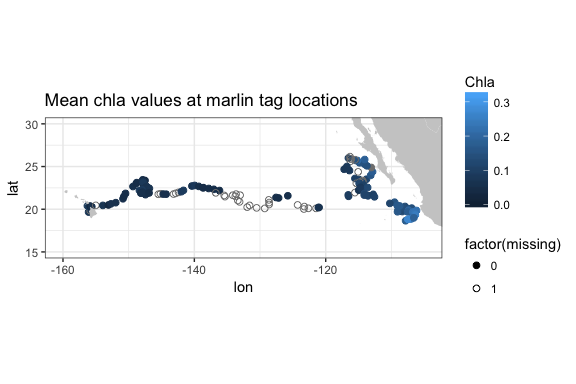### Comparing with the median

The `xtracto` routine extracts the environment data in a box (defined by `xlen` and `ylen`) around the given location, and calculates statistics of the data in that box. The median chla concentration may make more sense in this situation

``````require(ggplot2)
# plot using ggplot
z <- ggplot(alldata, aes(x = lon, y = lat)) +
geom_point(aes(colour = median, shape = factor(missing)), size = 2.) +
scale_shape_manual(values = c(19, 1))
z + geom_polygon(data = w, aes(x = long, y = lat, group = group), fill = "grey80") +
theme_bw() +
scale_colour_gradient(limits = c(0., 0.32), "Chla") +
coord_fixed(1.3, xlim = xlim, ylim = ylim) + ggtitle(" Median chla values at marlin tag locations")``````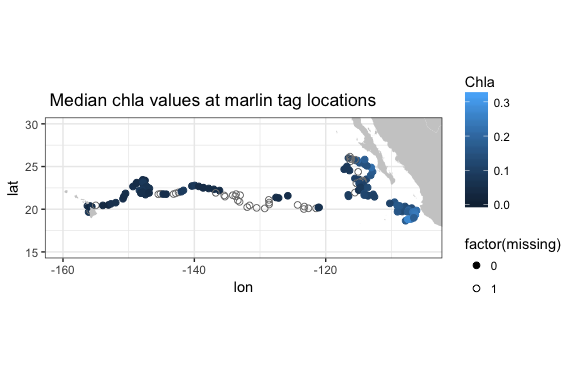The median chla is smaller than the mean chla (a maximum of 0.273 compared to 0.323).

### Topography data

In addition to satellite data, `xtractomatic` can access topographic data. As an example we extract and plot the water depth (bathymetry) associated with the trackline.

Get the topography data along the track:

``````require("xtractomatic")
require("ggplot2")
ylim <- c(15, 30)
xlim <- c(-160, -105)
topo <- xtracto(tagData\$lon, tagData\$lat, tagData\$date, "ETOPO360", .1, .1)``````

and plot the track:

``````require("ggplot2")
ylim <- c(15, 30)
xlim <- c(-160, -105)
alldata <- cbind(tagData, topo)
alldata\$lon <- alldata\$lon - 360
z <- ggplot(alldata, aes(x = lon, y = lat)) +
geom_point(aes(colour = mean), size = 2.) +
scale_shape_manual(values = c(19, 1))
z + geom_polygon(data = w, aes(x = long, y = lat, group = group), fill = "grey80") +
theme_bw() +
coord_fixed(1.3, xlim = xlim, ylim = ylim) + ggtitle("Bathymetry at marlin tag locations")``````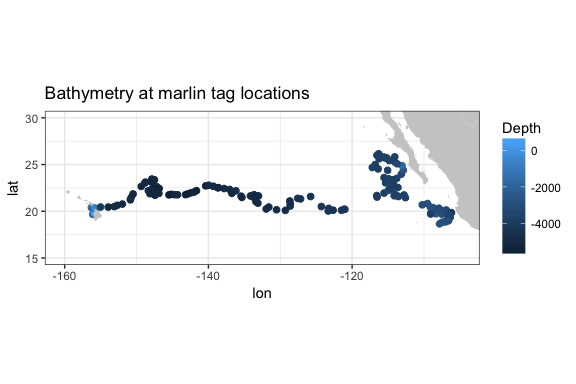The above example uses data already converted to R format, but clearly the utility of the `xtracto` function lies in using it with one’s own dataset. This dataset was read in from a text file called Marlin-tag38606.txt, which is also made available when the `xtractomatic` package is loaded. To be able to refer to this file:

``````
system.file("extdata", "Marlin-tag38606.txt", package = "xtractomatic")``````

The first 5 lines of the file can be viewed:

``````datafile <- system.file("extdata", "Marlin-tag38606.txt", package = "xtractomatic")

``````
Marlingtag38606 <- read.csv(datafile, head = TRUE, stringsAsFactors = FALSE, sep = "\t")
str(Marlingtag38606)
#> 'data.frame':    152 obs. of  7 variables:
#>  \$ date  : chr  "4/23/2003" "4/24/2003" "4/30/2003" "5/1/2003" ...
#>  \$ lon   : num  204 204 204 204 204 ...
#>  \$ lat   : num  19.7 19.8 20.4 20.3 20.3 ...
#>  \$ lowLon: num  204 204 204 204 204 ...
#>  \$ higLon: num  204 204 204 204 204 ...
#>  \$ lowLat: num  19.7 18.8 18.8 18.9 18.9 ...
#>  \$ higLat: num  19.7 20.9 21.9 21.7 21.7 ...``````

The file Marlingtag38606.txt is a “tab” separated file, not a comma seperated file, so the `sep` option in the `read.csv` function above indicates that to the function, and `stringsAsFactors = FALSE` tells the function not to treat the dates as factors.

To be useful the date field, now formatted for example as `4/23/2003`, needs to be converted to an R date field:

``Marlintag38606\$date <- as.Date(Marlintag38606\$date, format='%m/%d/%Y')``

The format of the date has to be given because the dates in the file are not in one of the formats recognized by `as.Date`. A small y (%y) indicates the year has 2 digits (97) while a capital Y (%Y) indicates the year has 4 digits (1997). If your file is in the EU format with commas as decimal points and semicolons as field separators then use read.csv2 rather than read.csv.

This dataset includes the range of the 95% confidence interval for both the latitude and the longitude, which are the last four columns of data. In the example above the search “radius” was read into xtracto was a constant value of 0.2 degrees, but a vector can also be read in. To use the limits given in the file, create the vectors as follows:

``````xrad <- abs(Marlintag38606\$higLon - Marlintag38606\$lowLon)

and then use these for the `xlen` and `ylen` inputs in `xtracto`:

``````xpos <- Marlintag38606\$lon
ypos <- Marlintag38606\$lat
tpos <- Marlintag38606\$date

## Using `xtracto_3D`

### Comparing chlorophyll estimates from different satellites

This example extracts a time-series of monthly satellite chlorophyll data for the period 1998-2014 from three different satellites ( SeaWIFS, MODIS and VIIRS ), using the `xtracto_3D` function. The extract is for an area off the coast of California. `xtracto_3D` extracts data in a 3D bounding box where `xpos` has the minimum and maximum longitude, `ypos` has the minimum and maximum latitude, and `tpos` has the starting and ending time, given as “YYY-MM-DD”, describing the bounding box.

First we define the longitude, latitude and temporal boundaries of the box:

``````xpos <- c(235, 240)
ypos <- c(36, 39)
tpos <- c('1998-01-01', '2014-11-30') ``````

The extract will contain data at all of the longitudes, latitudes and times in the requested dataset that are within the given bounds.

If we run the `xtracto_3D` using the full temporal contraints defined above for SeaWiFS we get an error:

``````require(xtractomatic)
chlSeaWiFS <- xtracto_3D(xpos, ypos, tpos, 'swchlamday')
#>  "tpos  (time) has elements out of range of the dataset"
#>  "time range in tpos"
#>  "1998-01-01,2014-11-30"
#>  "time range in ERDDAP data"
#>  "1997-09-16,2010-12-16T12:00:00Z"
#> Error in xtracto_3D(xpos, ypos, tpos, "swchlamday"): Coordinates out of dataset bounds - see messages above``````

The error occurs because the time-range specified is outside of the time-bounds of the requested dataset ( SeaWIFS data stopped in 2010). The error message generated by `xtracto_3D` explains this, and gives the temporal boundaries of the dataset. A simple way to extract to the end of a dataset is to use “last” (see Taking advantage of “last times”):

``````require(xtractomatic)
require(lubridate)
tpos <- c("1998-01-16","last")
SeaWiFS <- xtracto_3D(xpos, ypos, tpos, 'swchlamday')
SeaWiFS\$time <- as.Date(SeaWiFS\$time)``````
``````#> Loading required package: lubridate
#>
#> Attaching package: 'lubridate'
#> The following object is masked from 'package:base':
#>
#>     date``````

A similar extract can be made for the MODIS dataset. We know that the time-range of the MODIS dataset is also smaller than that defined by `tpos`. We can determine the exact bounds of the MODIS dataset by using the `getInfo` function:

``````require(xtractomatic)
getInfo('mhchlamday')
#>  "mhchlamday"
#>
#> dtypename                                                                                 mhchlamday
#> datasetname                                                                           erdMH1chlamday
#> longname          Chlorophyll-a, Aqua MODIS, NPP, L3SMI, Global, Science Quality (Monthly Composite)
#> varname                                                                                  chlorophyll
#> hasAlt                                                                                         FALSE
#> latSouth                                                                                       FALSE
#> lon360                                                                                         FALSE
#> minLongitude                                                                               -179.9792
#> maxLongitude                                                                                179.9792
#> longitudeSpacing                                                                          0.04166667
#> minLatitude                                                                                -89.97916
#> maxLatitude                                                                                 89.97918
#> latitudeSpacing                                                                          -0.04166667
#> minAltitude                                                                                     <NA>
#> maxAltitude                                                                                     <NA>
#> minTime                                                                                   2003-01-16
#> maxTime                                                                                   2016-11-16
#> timeSpacing(days)                                                                   30.4326241134752
#> infoURL                                                             http://oceancolor.gsfc.nasa.gov/``````

which gives us the min and max time of the dataset, which we can be used in the call (or use “last” as above):

``````require(xtractomatic)
require(lubridate)
tpos <- c('2003-01-16', "last")
MODIS <- xtracto_3D(xpos, ypos, tpos, 'mhchlamday')
MODIS\$time <- as.Date(MODIS\$time)``````

Similarly we can extract data from the VIIRS dataset.

``````require(xtractomatic)
require(lubridate)
tpos <- c("2012-01-15", "last")
VIIRS <- xtracto_3D(xpos, ypos, tpos, 'erdVH2chlamday')
VIIRS\$time <- as.Date(VIIRS\$time)``````

`xtracto_3d` returns a list of the form:

• extract\$data : num [longitude, latitude, time]
• extract\$varname : character string with the name of the variable
• extract\$datasetname: character string of the ERDDAP dataset ID
• extract\$latitude : num [latitude] latitudes of extract
• extract\$longitude : num [longitude] longitude of extact
• extract\$time : chr [time] times of extract
• extract\$altitude : num altitude of extract

in this case the varname is chla, the ERDDAP `datasetID` is erdVH2chlamday, and data contains the data on the grid defined by longitude, latitude and time. The data from the different satellites can be compared by mapping the values for a given time period. To do so we define a helper function `mapFrame` to reshape the data to be used in `ggplot2`.

``````mapFrame <- function(longitude, latitude, chla){
dims <- dim(chla)
chla <- array(chla, dims * dims)
longitude <- longitude - 360
chlaFrame <- expand.grid(x = longitude, y = latitude)
chlaFrame\$chla <- chla
return(chlaFrame)
}``````

and also define a helper function `plotFrame` to plot the data:

``````plotFrame <- function(chlaFrame, xlim, ylim, title, logplot = TRUE){
require(mapdata)
require(ggplot2)
require(RColorBrewer)
w <- map_data("worldHires", ylim = ylim, xlim = xlim)
myplot <- ggplot(data = chlaFrame, aes(x = x, y = y, fill = chla)) +
geom_raster(interpolate = FALSE) +
geom_polygon(data = w, aes(x = long, y = lat, group = group), fill = "grey80") +
theme_bw() + ylab("latitude") + xlab("longitude") +
coord_fixed(1.3, xlim = xlim, ylim = ylim)
if (logplot) {
my.col <- colorRampPalette(rev(brewer.pal(11, "RdBu")))(5.5)
myplot <- myplot + scale_fill_gradientn(colours = my.col, na.value = NA, limits = c(-2, 4.5)) +
ggtitle(title)
}else{
my.col <- colorRampPalette(rev(brewer.pal(11, "RdBu")))((diff(range(chlaFrame\$chla, na.rm = TRUE))))
myplot <- myplot + scale_fill_gradientn(colours = my.col, na.value = NA) +
ggtitle(title)
}
return(myplot)
}``````

We examine chlorophyll in June 2012 a period when VIIRS and MODIS both had data. Starting with VIIRS:

``````require(lubridate)
xlim <- c(235, 240) - 360
ylim <- c(36, 39)
ttext <- VIIRS\$time[month(VIIRS\$time) == 6 & year(VIIRS\$time) == 2012]
chlaFrame <- mapFrame(VIIRS\$longitude, VIIRS\$latitude, VIIRS\$data[, , month(VIIRS\$time) == 6 & year(VIIRS\$time) == 2012])
chlaPlot <- plotFrame(chlaFrame, xlim, ylim, paste("VIIRS chla", ttext), logplot = FALSE)
chlaPlot
#> Warning: Removed 4462 rows containing missing values (geom_raster).``````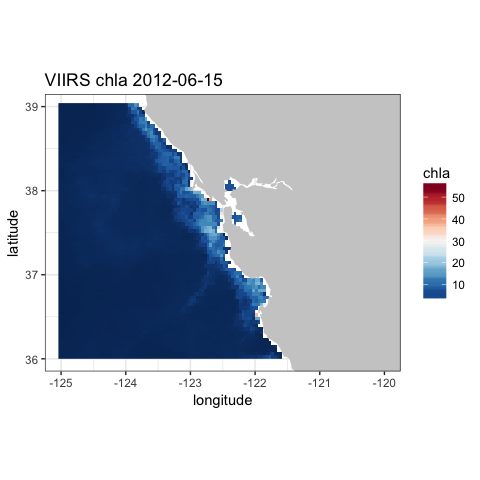Chlorophyll values are highly skewed, with low values most places and times, and then for some locations very high values. For this reason log(chlorophyll) is usually plotted.

``````xlim <- c(235, 240) - 360
ylim <- c(36, 39)
chlalogFrame <- mapFrame(VIIRS\$longitude, VIIRS\$latitude,
log(VIIRS\$data[, , month(VIIRS\$time) == 6 & year(VIIRS\$time) == 2012]))
chlalogPlot <- plotFrame(chlalogFrame, xlim, ylim, paste("VIIRS log(chla)", ttext))
chlalogPlot
#> Warning: Removed 4462 rows containing missing values (geom_raster).``````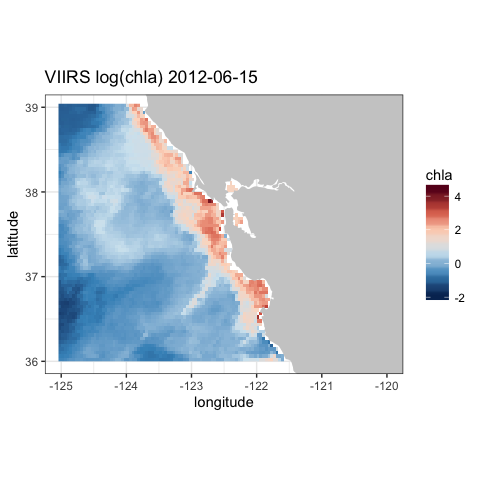And also for MODIS:

``````xlim <- c(235, 240) - 360
ylim <- c(36, 39)
ttext <- MODIS\$time[month(MODIS\$time) == 6 & year(MODIS\$time) == 2012]
chlalogFrame <- mapFrame(MODIS\$longitude, MODIS\$latitude,
log(MODIS\$data[, , month(MODIS\$time) == 6 & year(MODIS\$time) == 2012]))
chlalogPlot <- plotFrame(chlalogFrame, xlim, ylim, paste("MODIS log(chla)", ttext))
chlalogPlot
#> Warning: Removed 4502 rows containing missing values (geom_raster).``````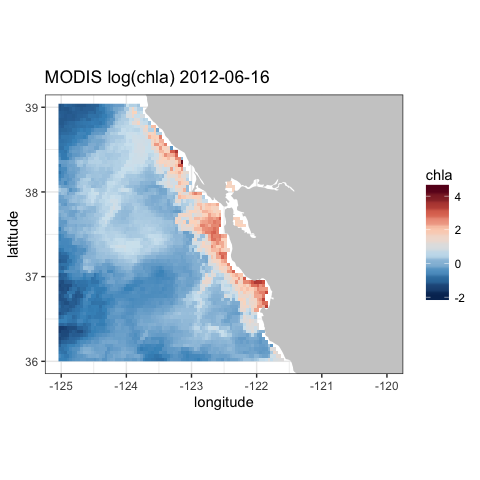SeaWiFS stopped functioning at the end of 2010, so we look at June, 2010:

``````xlim <- c(235, 240) - 360
ylim <- c(36, 39)
ttext <- SeaWiFS\$time[month(SeaWiFS\$time) == 6 & year(SeaWiFS\$time) == 2010]
chlalogFrame <- mapFrame(SeaWiFS\$longitude, SeaWiFS\$latitude,
log(SeaWiFS\$data[, , month(SeaWiFS\$time) == 6 & year(SeaWiFS\$time) == 2010]))
chlalogPlot <- plotFrame(chlalogFrame, xlim, ylim, paste("SeaWiFS log(chla)", ttext))
chlalogPlot
#> Warning: Removed 1133 rows containing missing values (geom_raster).``````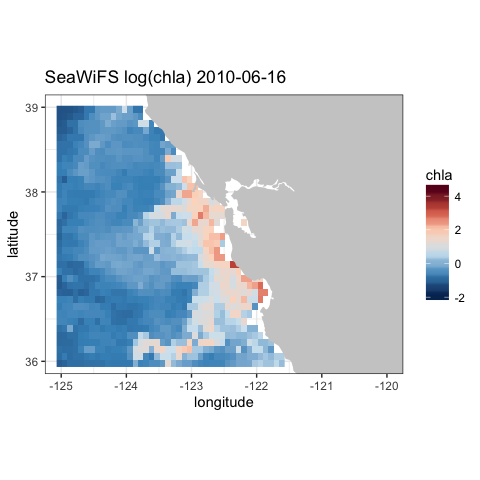We can also look at changes in log(chlorophyll) over time for a given region. We examine a higly productive region just north of San Francisco, with limits (38N, 38.5N) and (123.5W, 123W).

``````require(ggplot2)
require(mapdata)
xlim1 <- c(-123.5, -123) + 360
ylim1 <- c(38, 38.5)
w <- map_data("worldHires", ylim = c(37.5, 39), xlim = c(-123.5, -122.))
z <- ggplot() + geom_polygon(data = w, aes(x = long, y = lat, group = group), fill = "grey80") +
theme_bw() +
coord_fixed(1.3, xlim = c(-123.5, -122.), ylim = c(37.5, 39))
z + geom_rect(aes(xmin = -123.5, xmax = -123., ymin = 38., ymax = 38.5), colour = "black", alpha = 0.) +
theme_bw() + ggtitle("Location of chla averaging")``````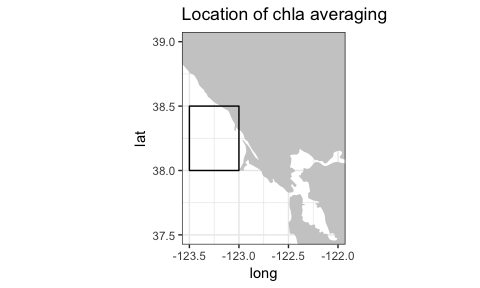We define a helper function `chlaAvg` which finds the latitudes and longitudes in the datasets that are closest to the given limits, extracts the subset that is within that region, and then averages over the region to create a time series.

``````chlaAvg <- function(longitude, latitude, chlaData, xlim, ylim, dStart){
xIndex <- xlim
yIndex <- ylim
yIndex <- which.min(abs(latitude - ylim))
yIndex <- which.min(abs(latitude - ylim))
xIndex <- which.min(abs(longitude - xlim))
xIndex <- which.min(abs(longitude - xlim))
chlaAvg <- apply(tempData, 3, mean, na.rm = TRUE)
chlaAvg <- ts(chlaAvg, frequency = 12, start = dStart)
return(chlaAvg)
}``````

We use `chlaAvg` to extract the three time series over the region, combine them and plot the result.

``````require(ggplot2)
require(ggfortify)
xlim1 <- c(-123.5, -123) + 360
ylim1 <- c(38, 38.5)
# Get both the average, and the average of log transformed chl for each point in the time series
SeaWiFSavg <- chlaAvg(SeaWiFS\$longitude, SeaWiFS\$latitude, SeaWiFS\$data, xlim1, ylim1,c(1998, 1))
SeaWiFSlog <- chlaAvg(SeaWiFS\$longitude, SeaWiFS\$latitude, log(SeaWiFS\$data), xlim1, ylim1, c(1998, 1))
# Run the same steps again for the MODIS and VIIRS datasets
MODISavg <- chlaAvg(MODIS\$longitude, MODIS\$latitude, MODIS\$data, xlim1, ylim1, c(2003, 1))
MODISlog <- chlaAvg(MODIS\$longitude, MODIS\$latitude, log(MODIS\$data), xlim1, ylim1, c(2003, 1))
# run the same for VIIRS
VIIRSavg <- chlaAvg(VIIRS\$longitude, VIIRS\$latitude, VIIRS\$data, xlim1, ylim1, c(2012, 1))
VIIRSlog <- chlaAvg(VIIRS\$longitude, VIIRS\$latitude, log(VIIRS\$data), xlim1, ylim1, c(2012, 1))``````

First the raw chla values:

``````require(ggfortify)
Chla <- cbind(VIIRSavg, MODISavg, SeaWiFSavg)
autoplot(Chla, facets = FALSE, na.rm = TRUE) + theme_bw() + ggtitle("Chla time series off Point Reyes")
#> Warning: Removed 312 rows containing missing values (geom_path).``````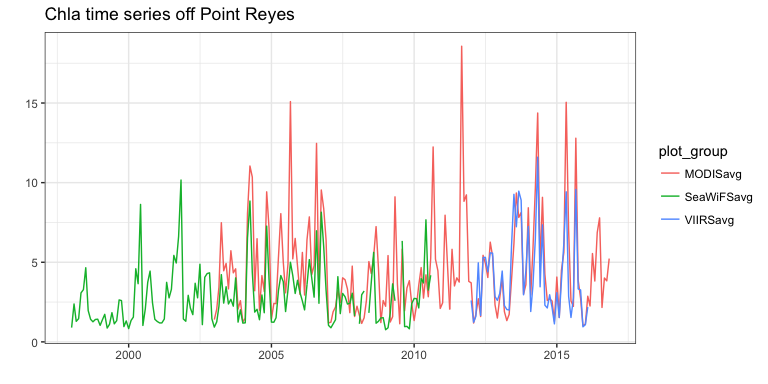and then log(chla):

``````require(ggfortify)
logChla <- cbind(VIIRSlog, MODISlog, SeaWiFSlog)
autoplot(logChla, facets = FALSE, na.rm = TRUE) + theme_bw() + ggtitle("log(Chla) time series off Point Reyes")
#> Warning: Removed 312 rows containing missing values (geom_path).``````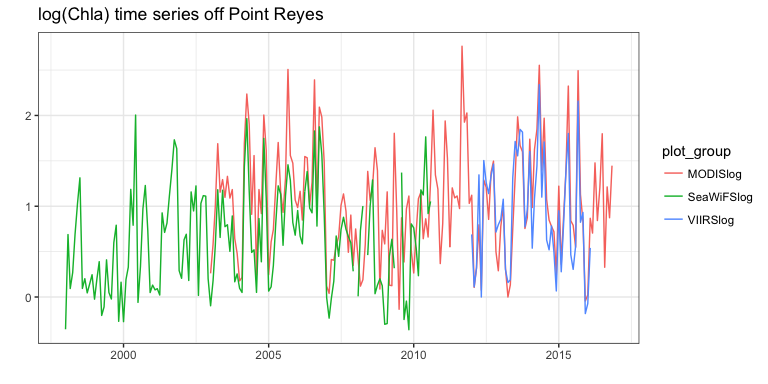### Upwelling

ERD for many years has produced 6-hourly Bakun upwelling indices at 15 set locations. But suppose you want the values at a different location? To do this we define a function `upwell` that rotates Ekman transport values to obtain the offshore component (upwelling index), and also define a function `plotUpwell` to plot upwelling. `xtracto_3D` can download Ekman transport calculated from Fleet Numerical Meteorlogical and Oceanographic Center (FNMOC) pressure fields:

``````upwell <- function(ektrx, ektry, coast_angle){
pi <- 3.1415927
alpha <- (360 - coast_angle) * degtorad
s1 <- cos(alpha)
t1 <- sin(alpha)
s2 <- -1*t1
t2 <- s1
perp <- s1 * ektrx + t1 * ektry
para <- s2 * ektrx + t2 * ektry
return(perp/10)
}``````
``````plotUpwell <- function(upwelling, upDates){
require(ggplot2)
require(ggfortify)
require(xts)
temp <- xts(upwelling, order.by = upDates)
autoplot(temp) + theme_bw() + ylab("Upwelling")
}``````

We calculate 6-hourly upwelling at (37,-122) for the year 2005 and use the coast angle that ERD uses for (36N , 122W) which is 152 degrees.

``````require(xtractomatic)
xpos <- c(238, 238)
ypos <- c(37, 37)
tpos <- c("2005-01-01", "2005-12-31")
ektrx <- xtracto_3D(xpos, ypos, tpos, "erdlasFnTran6ektrx")
ektry <- xtracto_3D(xpos, ypos, tpos, "erdlasFnTran6ektry")
upwelling <- upwell(ektrx\$data, ektry\$data, 152)``````

A plot of the result:

``````plotUpwell(upwelling, as.Date(ektrx\$time))
#>
#> Attaching package: 'zoo'
#> The following objects are masked from 'package:base':
#>
#>     as.Date, as.Date.numeric``````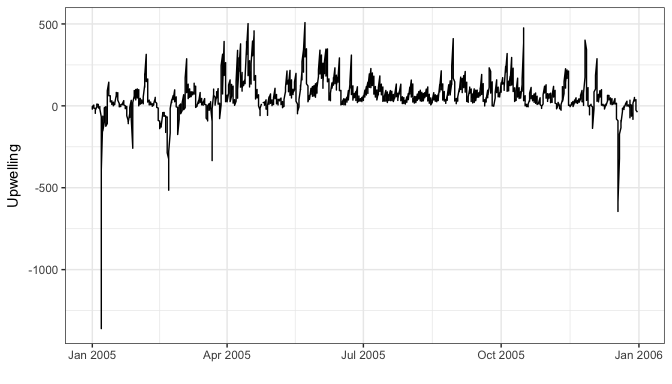The one very low downwelling value distorts the plot, so values < -500 are set to NA and the data are replotted:

``````tempUpw <- upwelling
tempUpw[tempUpw < -500] <- NA
plotUpwell(tempUpw, as.Date(ektrx\$time))``````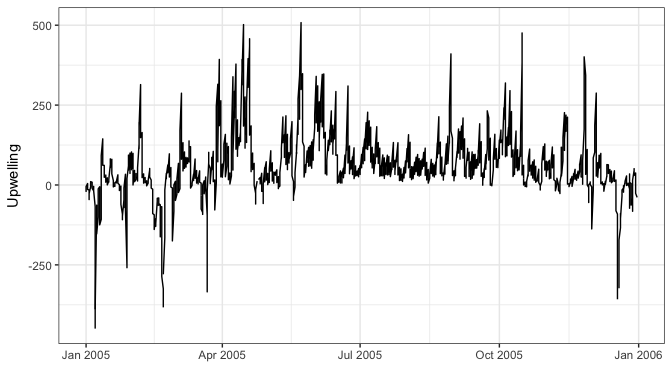In 2005 there was a large die-off of sea animals and many felt that is was due to an anomalously delayed upwelling. Let’s compare upwelling in 2005 at that location with upwelling in 1977:

``````require(xtractomatic)
tpos <- c("1977-01-01", "1977-12-31")
ektrx77 <- xtracto_3D(xpos, ypos, tpos, "erdlasFnTran6ektrx")
ektry77 <- xtracto_3D(xpos, ypos, tpos, "erdlasFnTran6ektry")
upwelling77 <- upwell(ektrx77\$data, ektry77\$data, 152)
# remove the one big storm at the end
tempUpw <- upwelling77
tempUpw[tempUpw < -500] <- NA
plotUpwell(tempUpw, as.Date(ektrx77\$time))``````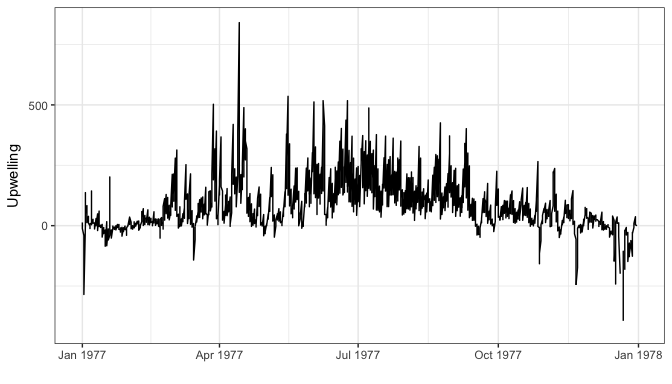While we have not looked at all areas along the coast, at least for this location it is difficult to see upwelling in 2005 as being any more delayed than in 1977 when there was not the same affect on animals.

### Comparisons of Wind Stress

A problem faced when using satellite based estimates of wind stress is that the QuikSCAT based estimates start in 1999-07-21 and end in 2009-11-19, while the ASCAT based estimates start in 2009-10-03 and are ongoing. A comparison of the data over the period of overlap would be of interest.

We examine north-south wind stress (tauy) over the period of overlap at (36N, 121W) (we go a little more offshore because the satellite data is not resolved close to the coast), and compare 3-day composites of tauy. In this region tauy is the main component of upwelling as the coastline runs almost north-south and the winds are mostly from the north or north-west.

``````require(xtractomatic)
xpos <- c(237, 237)
ypos <- c(36, 36)
tpos <- c("2009-10-04", "2009-11-19")
ascat <- xtracto_3D(xpos, ypos, tpos, "erdQAstress3daytauy")
quikscat <- xtracto_3D(xpos, ypos, tpos, "erdQSstress3daytauy")``````

Plotting the result

``````require(ggplot2)
require(ggfortify)
require(xts)
ascatStress <- xts(ascat\$data, order.by = as.Date(ascat\$time))
quikscatStress <- xts(quikscat\$data, order.by = as.Date(quikscat\$time))
stressCompare <- cbind(ascatStress,quikscatStress)
colnames(stressCompare) <- c("ascat", "quikscat")
autoplot(stressCompare, facets = FALSE) + theme_bw()``````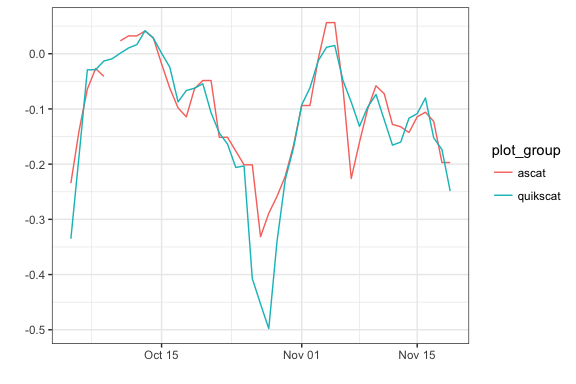While the two wind stress series are close, there is a significant difference in the negative (southward) value on October 28, that is there will be a signifcant difference in the estimated (positive) upwelling value. Combining the series into a longer series would be desirable, but this suggests that there could be problems with inter-year comparisons due to the different sources of the data.

### Taking advantage of “last times”

The ERDDAP server understands a time of “last” as being the last time period available for that dataset, and permits arithmetic using “last”, that is “last-5” is a valid time and will be 5 time periods before the last time (note that since different datasets have different time periods this difference is dataset dependent). For example to extract the last six months of SST from POES AVHRR we look at the monthly agsstmday dataset:

``````require(xtractomatic)
xpos <- c(235, 240)
ypos <- c(36, 39)
tpos <- c("last-5", "last")
poes <- xtracto_3D(xpos, ypos, tpos, "agsstamday")``````

Plotting the result using faceting in ggplot2:

``````require(ggplot2)
require(reshape2)
require(mapdata)

melt_sst <- function(longitude, latitude, mTime, sst) {
dimnames(sst) <- list(long = longitude, lat = latitude)
ret <- melt(sst, value.name = "sst")
cbind(date = mTime, ret)
}
xpos <- xpos - 360
longitude <- poes\$longitude - 360
latitude <- poes\$latitude
imon <- 1:5
tmp <- lapply(imon, function(x) melt_sst(longitude, latitude, poes\$time[x], poes\$data[,,x]))
allmons <- do.call(rbind, tmp)
w <- map_data("worldHires", ylim = ypos, xlim = xpos)
ggplot(data = allmons, aes(x = long, y = lat, fill = sst)) +
geom_polygon(data = w, aes(x = long, y = lat, group = group), fill = "grey80") +
geom_raster(interpolate = TRUE) +
scale_fill_gradientn(colours = rev(rainbow(10)), na.value = NA) +
theme_bw() +
coord_fixed(1.3,xlim = xpos, ylim = ypos) +
facet_wrap(~ date, nrow = 2)
#> Warning: Removed 4070 rows containing missing values (geom_raster).``````